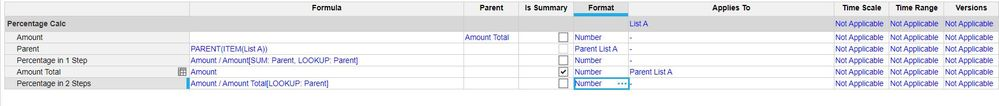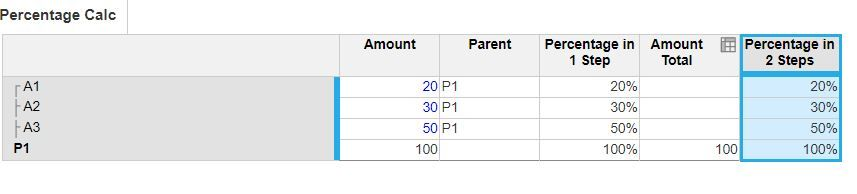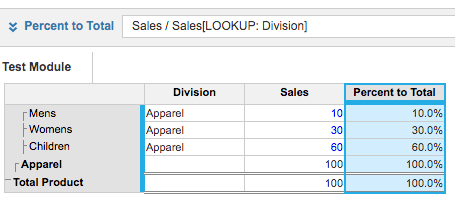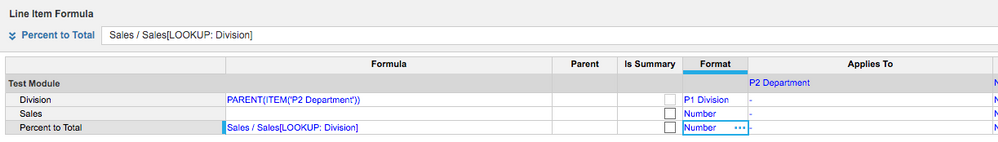# percentages of a lineHi:

Apologies for the simple question but we are new at this!!!

I need to work out how to work out the % of a item in a line.

Using an excel equation it will be sometime like - a2/sum(a2:a5)

I am sure it is something very straight forward but having no joy so far!!

Many thanks for the help in advance!!

Tagged:

•Hi,

I have created a simple example with a list "List A" that rolls up to the list "Parent List A", proposing two different approaches:

- Percentage in 1 step using the SUM & LOOKUP

- Percentage in 2 steps breaking the SUM & LOOKUP in two steps with the help of an extra line item "Amount Total" (Suggested approachBlueprintGrid View

I hope this helps

Regards,

Antonio

•@a.dilieto is right.

Just try to avoid line items that use sum and lookup in the same formula. These are not very efficient formulas.

If you use the DISCO methodology, which is highly recommended, the parent lookup should be in a system module. "Calculate once", "Reuse Often".

In this example "division" is in the same module but really belongs in a system module.•If you are needing a the sum to be dynamic (say three months forward), then MOVINGSUM is good for that.

Also, if it is the year end number you want, use a time settings module that holds, say FY2019, as a formatted line item

You can then write

line item/line item[LOOKUP:Time Settings.Lookup value]

I hope that helps

David